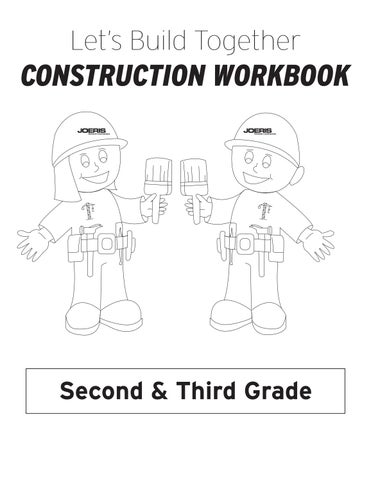Letâ&amp;#x20AC;&amp;#x2122;s Build Together

CONSTRUCTION WORKBOOK

Directions: Look at the blueprint and blueprint key. Use them to answer the questions below.

Blueprint Key Outside Wall

Inside Wall

Doors

Window

Carpet

1. How many doors are there? _________ 2. Color the rooms that have carpet green. How many rooms have carpet? _________ 3. True or False, there are four doors that lead outside? _________ 4. Color the windows blue. How many windows are there? _________

Draw a School House!

Use as many shapes as you want but you MUST include:

oval

rectangle

triangle

trapezoid

star

Color by the Number!

Solve the problems and pick the right color. 5+4=

5+6= 3 +6

9 +2

8 +3

5 +5

3+7=

7+8=

6+4=

5+9=

9+9=

6+7= 9+6=

2 +8

7+7=

1+9=

7 +6

7+8=

8 +6

9+6=

2+8= 8+7= 7+3=

4+6=

1 +7

9+5=

7 +7

4 +4

2 +2

2+1=

9+9=

9 +5

5+8=

9 +7

7+4=

1+1= 9+1=

8+8=

5 +5

8+1= 8+6=

6 +1

5+3= 5 +8 2 +3

6+6=

5 +2

3+1= 8 +6

7+5=

5+1=

9+8= 4 +8

2+4=

Blue: 10,13,14,15,18

Green: 4,7

Orange: 9,11

Purple: 8,17

Red: 2,3,16

Brown: 5,6,12

3 +9

To, Too and Two

Directions: Read each sentence and write to, too or two in order to make the sentence correct. Color the tool each time you use the word. two

to

to

two

too

to

to

too

to

too

1. Kate and Sam are going _____ the hardware store. 2. Sally want to go, _____. 3. They want _____ buy tools at the hardware store. 4. The tools cost _____ dollars each. 5. Kate and Sam ride their bikes _____ the hardware store. 6. They forgot _____ pick up Sally. 7. It only took _____ minutes to ride to Sallyâ&amp;#x20AC;&amp;#x2122;s house. 8. Sally wants to get tools, _____. 9. They had _____ much fun, and agreed _____ go for a ride tomorrow. Write your own sentence using the word to.

1. _________________________________________________________ Write your own sentence using the word too.

2. _________________________________________________________ Write your own sentence using the word two.

3. _________________________________________________________

Construction

Conjunctions!

Directions: Circle the conjuration that finishes the sentence. Write in the blank.

1. I bring my hammer ______ nails to work. so

because

and

2. I wear a hardhat ______ my head will be safe. or

so

but

3. My friends ______ I work together. and

but

because

4. We like to build things ______ it is fun. but

because

and

WORD SEARCH!

Find the words in the puzzle. Words are hidden and .

D C L T M K S N C L J F

Z W A L L O T S A R X G

H G U R G E E U R M J F

A J B A H T E G P K W O

R C B U S A L K E M J I

D L O C I E M C T Q P Z

H B W N K L H M B K G J

A M L R C S D W E Q K T

T D A U E R A I P R E O

N R O T E N E F N B C O

A C O U E P C T E G L L

I R O O T R R H E T U S

L A U S F I I I A G Y V

B N I N C L N A N X F L

K E B B B R O V L T G B

F E N C E O E S W S C A

WORD BANK BEAM BUILDING CRANE HARDHAT ROOF STEEL WRENCH

BLUEPRINT CARPET FENCE MATERIALS SAFETY TOOLS

BOLT CONCRETE HAMMER NAIL SCREW WALL

C Z P N B A L W S J A T

G H E G Y Q M T R C J U

CROSSWORD PUZZLE! Word Bank Wall Hammer Dirt Nail Paint

Floor Wood Window Screw Roof

2

Tools Fence Door Drill

1

3 5

4

6

7

8 10

11

ACROSS 1. Separates one room from another 4. Opposite of a drill 5. Found outside on the ground 7. Opposite of a nail 10. Where carpet and tile are 11. Opposite of a screw

DOWN 1. You look out of it 2. The color on the wall 3. Keeps people out 6. Top of a building 8. Used to build things 9. Opposite of a hammer

9

Finish Building the School! Practice in Symmetry: Use the grid to draw a mirror image of the Schoolâ&amp;#x20AC;&amp;#x2122;s left side on the right side of the axis.